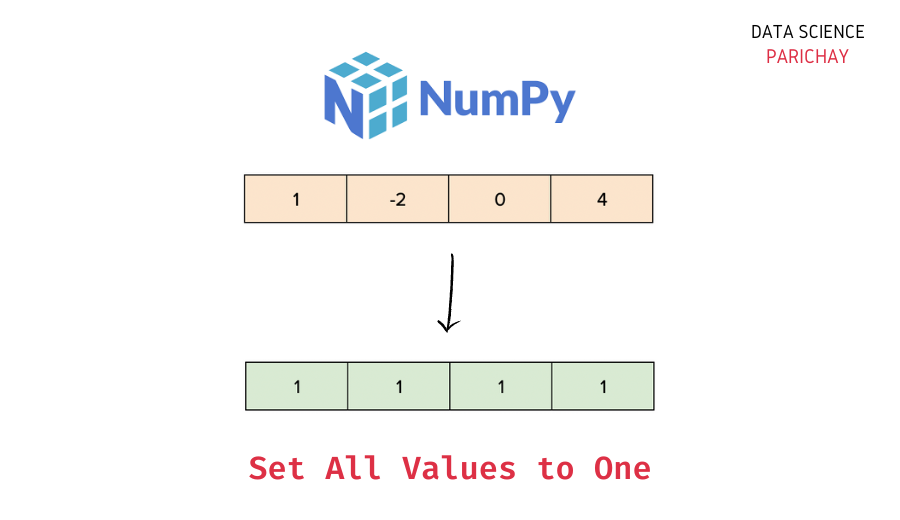# Numpy – Set All Values to One in Array

The Numpy library in Python comes with a number of built-in functions to manipulate the data in arrays. In this tutorial, we will look at a function that helps us set all the values in a Numpy array to one.

## How to set all values to one in Numpy?

You can use the `numpy.ndarray.fill()` function to set all the values in a Numpy array to one. Pass `1` as the argument (this is the value used to fill all the values in the array).

The following is the syntax –

```# set all values in numpy array ar to 1
ar.fill(1)```

It modifies the array in-place, filling each value with one (the passed value).

Let’s now look at a step-by-step example of using the above function.

### Step 1 – Create a Numpy array

First, we will create a Numpy array that we will use throughout this tutorial.

```import numpy as np

# create a numpy array
ar = np.array([-2, -1, 0, 1, 2, 3, -4])
# display the array
print(ar)```

Output:

`[-2 -1  0  1  2  3 -4]`

Here, we used the `numpy.array()` function to create a Numpy array containing some numbers.

📚 Data Science Programs By Skill Level

Introductory

Intermediate ⭐⭐⭐

🔎 Find Data Science Programs 👨‍💻 111,889 already enrolled

Disclaimer: Data Science Parichay is reader supported. When you purchase a course through a link on this site, we may earn a small commission at no additional cost to you. Earned commissions help support this website and its team of writers.

### Step 2 – Set each value to 1 using `numpy.ndarray.fill()`

Apply the `numpy.ndarray.fill()` function on the array and pass `1` as the parameter to set each value to one in the array.

Let’s apply this function to the array created above.

```# set all values to one
ar.fill(1)
# display the array
print(ar)```

Output:

`[1 1 1 1 1 1 1]`

You can see that each value in the array `ar` is now 1.

The `numpy.ndarray.fill()` function works similarly on higher-dimensional arrays. For example, let’s apply this function to a 2D array of some numbers.

```# create 2D numpy array
ar = np.array([[-1, 2, -3],
[0, 51, 7],
[1, 125, 8]])
# set all values to one
ar.fill(1)
# display the array
print(ar)```

Output:

```[[1 1 1]
[1 1 1]
[1 1 1]]```

You can see that each value in the above 2D array is now one.

You might also be interested in –

•